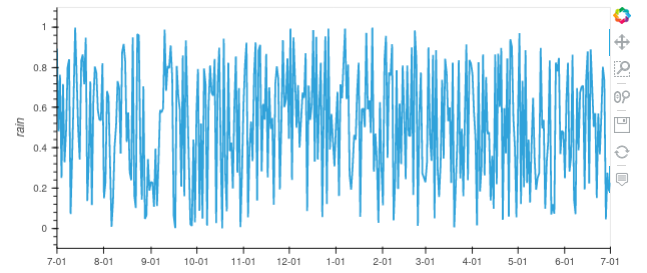# How to set xticks for data of string type (categorical) with hvPlot

I have a dataframe `region_cumulative_df_sel` as below:

``````Month-Day  regions  RAIN_PERCENTILE_25  RAIN_PERCENTILE_50  RAIN_PERCENTILE_75  RAIN_MEAN   RAIN_MEDIAN
07-01      1        0.0611691028        0.2811064720        1.9487996101    1.4330813885    0.2873695195
07-02      1        0.0945720226        0.8130480051        4.5959815979    2.9420840740    1.0614821911
07-03      1        0.2845511734        1.1912839413        5.5803232193    3.7756001949    1.1988518238
07-04      1        0.3402922750        3.2274529934        7.4262523651    5.2195668221    3.2781836987
07-05      1        0.4680584669        5.2418060303        8.6639881134    6.9092760086    5.3968687057
07-06      1        2.4329853058        7.3453550339        10.8091869354   8.7898645401    7.5020875931
... ...
... ...
... ...
06-27      1        382.7809448242      440.1162109375      512.6233520508  466.4956665039  445.0971069336
06-28      1        383.8329162598      446.2222900391      513.2116699219  467.9851379395  451.1973266602
06-29      1        385.7786254883      449.5384826660      513.4027099609  469.5671691895  451.2281188965
06-30      1        386.7952270508      450.6524658203      514.0201416016  471.2863159180  451.2484741211
``````

The index “Month-Day” is a type of String indicating the first day and the last day of a calendar year (from 1st of July to the 30th of June of the next year) instead of type of datetime.

I need to use hvPlot to develop an interactive plot. I tried the following code:

region_cumulative_df_sel.hvplot(width=900)

It is hard to view the labels on the x axis. How can change the xticks to show only 1st of each month, e.g. “07-01”, “08-01”, “09-01”, … …, “06-01”?

1 Like

The way I would do it is to convert `Month-Day` to datetime with an arbitrary year and then use custom models to hide the year.

``````import hvplot.pandas
import numpy as np
import pandas as pd

index = pd.date_range("2020-07-01", "2021-07-01", freq="D")
df = pd.DataFrame(np.random.random(index.size), index=index, columns=["rain"])

# Using custom models
from bokeh.models import DatetimeTickFormatter, HoverTool

# https://docs.bokeh.org/en/2.4.1/docs/reference/models/formatters.html#datetimetickformatter
tickfmt = DatetimeTickFormatter(years="%m-%d", months="%m-%d")

tooltips = [
("Month-Day", "@index{%m-%d}"),
("rain", "@rain"),
]
hover = HoverTool(tooltips=tooltips, formatters={"@index": "datetime"})

df.hvplot(xformatter=tickfmt, tools=[hover])
``````
1 Like

Just wanted to add that if you turn your date index into a real date type, hvplot will automatically make the xaxis look nice without anything further needed (I’m not sure how important it is for @achen to have the xtick actually just ‘M-D’ format or not).

2 Likes

Thank you @Hoxbro so much for the promptly reply!

Your code worked pretty well. I have slightly made some changes.

``````# DEAL WITH DATE
region_cumulative_df_sel['Month-Day-2'] = pd.to_datetime( Region_cumulative_df_sel['Month-Day'],format="%m-%d")  ##Convert to datetime

# ADD ONE YEAR TO THE ARBITRARY YEAR IF DATE IS BETWEEN 01-Jan and 30-June FOR PLOTTING
region_cumulative_df_sel['Month-Day-3'] = np.where(pd.DatetimeIndex(region_cumulative_df_sel['Month-Day-2']).month < 7, region_cumulative_df_sel['Month-Day-2'] + pd.DateOffset(years=1), region_cumulative_df_sel['Month-Day-2'])

# Remove old String-type "Month-Day" and "Month-Day-2"
region_cumulative_df_sel= region_cumulative_df_sel.drop(columns=['Month-Day', 'Month-Day-2'])

# STACK AND RESHAPE THE COLUMNS AND ROWS
df_csv_concat_stack = (region_cumulative_df_sel.set_index(["Month-Day-3", "regions"])
.stack()
.reset_index(name='Rainfall')
.rename(columns={'level_2':'Variable'}))
``````
``````## Define widgets
name='Region',
options=list(np.unique(df_csv_concat_stack.regions)),
button_type='success'
)

## Define a Dataframe pipeline
ipipeline = (
idf[
idf.regions == regions
].set_index('Month-Day-3')
)

## Here, I basically copied @Hoxbro code.
# Using custom models
from bokeh.models import DatetimeTickFormatter, HoverTool

# https://docs.bokeh.org/en/2.4.1/docs/reference/models/formatters.html#datetimetickformatter
tickfmt = DatetimeTickFormatter(years="%m-%d", months="%m-%d")

tooltips = [
("Month-Day", "@index{%m-%d}"),
("rain", "@Rainfall"),
]

hover = HoverTool(tooltips=tooltips, formatters={"@index": "datetime"})

ihvplot = ipipeline.hvplot.line(x='Month-Day-3', xformatter=tickfmt, y='Rainfall', by='Variable', tools=[hover], ylim=(0,800), line_width=3, height=900)
ihvplot
``````

The interactive plotting was generated pretty well except for the tooltips on hover. The “Month-Day” displayed “???”. How can I fix it?

Also the xticks show every other month e.g. “7-01” “9-01” … … “5-01” (M-D). How can I show every single month instead e.g. “7-01” “8-01” … … “6-01” (M-D)?

Thanks @droumis .
Yes it is very important to have xtick show actually the “M-D” format, so I need to remove the “Y” component and also show the “M-D” format in the tooltips on mouse hover as well.

1 Like

The interactive plotting was generated pretty well except for the tooltips on hover. The “Month-Day” displayed “???”. How can I fix it?

You should rename `@index` to the column name like this `@Month-Day-3` (or whatever it is). Both in tooltips and formatters.

Also the xticks show every other month e.g. “7-01” “9-01” … … “5-01” (M-D). How can I show every single month instead e.g. “7-01” “8-01” … … “6-01” (M-D)?

This could be done with MonthTicker, though it would be fixed to the month and not updating when zooming in.

``````from bokeh.models import DatetimeTickFormatter, HoverTool, MonthsTicker
...
df.hvplot(xformatter=tickfmt, tools=[hover], xticks=MonthsTicker(months=list(range(1, 13))))
``````

Alternative `DatetimeTicker` can be used, which will automatically update when zooming in, but will not always show each month.

``````from bokeh.models import DatetimeTickFormatter, HoverTool, DatetimeTicker
...
df.hvplot(xformatter=tickfmt, tools=[hover], xticks=DatetimeTicker(desired_num_ticks=12))
``````1 Like

The `xticks=DatetimeTicker(desired_num_ticks=12)` worked for me. Here is the result:

When zooming in, the xticks get correctly updated, which was what I wanted to achieve.

Many thanks @Hoxbro !